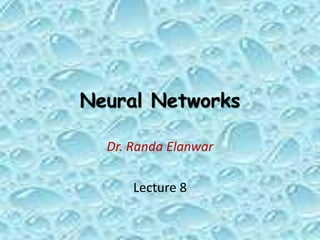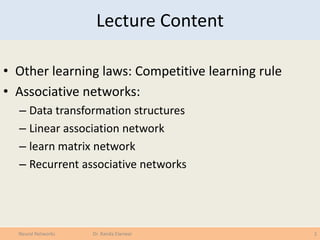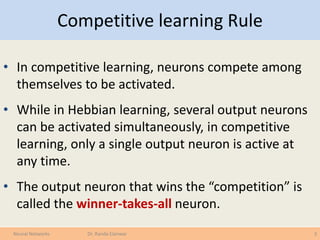Successfully reported this slideshow.

# Introduction to Neural networks (under graduate course) Lecture 8 of 9×

1 of 27 Ad
1 of 27 Ad

# Introduction to Neural networks (under graduate course) Lecture 8 of 9

Introduction and a historical review
Neural network concepts
Basic models of ANN
Linearly separable functions
Non Linearly separable functions
NN Learning techniques
Associative networks
Mapping networks
Spatiotemporal Network
Stochastic Networks

Introduction and a historical review
Neural network concepts
Basic models of ANN
Linearly separable functions
Non Linearly separable functions
NN Learning techniques
Associative networks
Mapping networks
Spatiotemporal Network
Stochastic Networks

## More Related Content

### Introduction to Neural networks (under graduate course) Lecture 8 of 9

1. 1. Neural Networks Dr. Randa Elanwar Lecture 8
2. 2. Lecture Content • Other learning laws: Competitive learning rule • Associative networks: – Data transformation structures – Linear association network – learn matrix network – Recurrent associative networks 2Neural Networks Dr. Randa Elanwar
3. 3. Competitive learning Rule • In competitive learning, neurons compete among themselves to be activated. • While in Hebbian learning, several output neurons can be activated simultaneously, in competitive learning, only a single output neuron is active at any time. • The output neuron that wins the “competition” is called the winner-takes-all neuron. 3Neural Networks Dr. Randa Elanwar
4. 4. Competitive learning Rule • Initially the weights in each neuron are random • Input values are sent to all the neurons • The outputs of each neuron are compared • The “winner” is the neuron with the largest output value • Having found the winner, the weights of the winning neuron are adjusted 4Neural Networks Dr. Randa Elanwar
5. 5. Competitive learning Rule • Weights are adjusted according to the following formula: • The learning coefficient  starts with a value of 1 and gradually reduces to 0. • This has the effect of making big changes to the weights initially, but no changes at the end. • The competitive learning rule defines the change Dwij applied to synaptic weight wij as 5Neural Networks Dr. Randa Elanwar     D ncompetitiothelosesneuronif,0 ncompetitiothewinsneuronif),( j jwx w iji ij 
6. 6. Competitive learning Rule • The overall effect of the competitive learning rule resides in moving the synaptic weight vector Wj of the winning neuron j towards the input pattern X. The matching criterion is equivalent to the minimum Euclidean distance between vectors. • The Euclidean distance between a pair of n-by-1 vectors X and Wj is defined by where xi and wij are the ith elements of the vectors X and Wj, respectively. 6Neural Networks Dr. Randa Elanwar 2/1 1 2 )(            n i ijij wxd WX
7. 7. Competitive learning Rule • To identify the winning neuron, jX, that best matches the input vector X, we may apply the following condition: where m is the number of neurons in the output layer. 7Neural Networks Dr. Randa Elanwar ,j j minj WXX  j = 1, 2, . . .,m
8. 8. Competitive learning Rule • Example: Suppose, for instance, that the 2- dimensional input vector X is presented to the three-neuron network, • The initial weight vectors, Wj, are given by 8Neural Networks Dr. Randa Elanwar        12.0 52.0 X        81.0 27.0 1W        70.0 42.0 2W        21.0 43.0 3W
9. 9. Competitive learning Rule • We find the winning (best-matching) neuron jX using the minimum- distance Euclidean criterion: • Neuron 3 is the winner and its weight vector W3 is updated according to the competitive learning rule. 9Neural Networks Dr. Randa Elanwar 2 212 2 1111 )()( wxwxd  73.0)81.012.0()27.052.0( 22  2 222 2 1212 )()( wxwxd  59.0)70.012.0()42.052.0( 22  2 232 2 1313 )()( wxwxd  13.0)21.012.0()43.052.0( 22  0.01)43.052.0(1.0)( 13113 D wxw 0.01)21.012.0(1.0)( 23223 D wxw
10. 10. Competitive learning Rule • The updated weight vector W3 at this iteration is determined as: • The weight vector W3 of the wining neuron 3 becomes closer to the input vector X with each iteration. 10Neural Networks Dr. Randa Elanwar                    D 20.0 44.0 01.0 0.01 21.0 43.0 )()()1( 333 ppp WWW        12.0 52.0 X
11. 11. Neural Processing 11Neural Networks Dr. Randa Elanwar •So far we have studied the NN structure, learning techniques, and problem solution methods from the mathematical point of view. In other words, how to solve a modeled problem but we still don’t know much about the physical problem itself. •NN are used to solve problems like: •Signal processing •Pattern recognition, e.g. handwritten characters or face identification. •Diagnosis or mapping symptoms to a medical case. •Speech recognition •Human Emotion Detection •Educational Loan Forecasting and much more
12. 12. Neural Processing • The common target in all these problems is that we need an intelligent tool (NN) that can learn from examples and perform data classification and prediction. • What is Classification? • The goal of data classification is to organize and categorize data in distinct classes. – A model is first created based on the data distribution. – The model is then used to classify new data. – Given the model, a class can be predicted for new data. • Classification = prediction for discrete values 12Neural Networks Dr. Randa Elanwar
13. 13. Neural Processing • Required classification is either: • Supervised Classification = Classification – We know the class labels and the number of classes • Unsupervised Classification = Clustering – We do not know the class labels and may not know the number of classes • What is Prediction? • The goal of prediction is to forecast or deduce the value of an attribute based on values of other attributes. – A model is first created based on the data distribution. – The model is then used to predict future or unknown values 13Neural Networks Dr. Randa Elanwar
14. 14. Neural Processing • The learning process leads to memory formation since it associates certain inputs to their corresponding outputs (responses) through weight adaptation. • The classification process uses the trained network to find out the responses corresponding to the new (unknown inputs). • Recall:- processing phase for a NN and its objective is to retrieve the information. The process of computing o for a given x (i.e. memory association) 14Neural Networks Dr. Randa Elanwar
15. 15. Neural Processing • The function of an associative memory is to recognize previously learned input vectors, even in the case where some noise has been added. • In other words, Associative Memory means accessing (Retrieving data out of) memory according to the content of the pattern (associated info) to get a response. 15Neural Networks Dr. Randa Elanwar
16. 16. Associative networks • Associative networks are types of neural networks with recurrent (feed back) connections used for pattern association. • We can distinguish between three overlapping kinds of associative networks: – Heteroassociative networks – Autoassociative networks – Pattern recognition/classification networks 16Neural Networks Dr. Randa Elanwar
17. 17. Associative networks • Heteroassociative Networks: 17Neural Networks Dr. Randa Elanwar
18. 18. Associative networks • Heteroassociative Networks: 18Neural Networks Dr. Randa Elanwar • Associations between pairs of patterns are stored • Distorted input pattern may cause correct heteroassociation at the output
19. 19. Associative networks • Autoassociative Networks: 19Neural Networks Dr. Randa Elanwar
20. 20. Associative networks • Autoassociative Networks: 20Neural Networks Dr. Randa Elanwar • Set of patterns can be stored in the network • If a pattern similar to a member of the stored set is presented, an association with the input of closest stored pattern is made
21. 21. Associative networks • Recognition/Classification Networks: 21Neural Networks Dr. Randa Elanwar
22. 22. Associative networks • Recognition/Classification Networks: 22Neural Networks Dr. Randa Elanwar • Set of input patterns is divided into a number of classes or categories • In response to an input pattern from the set, the classifier is supposed to recall the information regarding class membership of the input pattern.
23. 23. Data Transformation • Before classification data has to be prepared • Data transformation: – Discretization of continuous data – Normalization to [-1..1] or [0..1] • Data Cleaning: – Smoothing to reduce noise • Relevance Analysis: – Feature selection to eliminate irrelevant attributes • We finally get patterns/points/samples in the feature space that represent out data 23Neural Networks Dr. Randa Elanwar
24. 24. linear associative networks • The problem is known as linear if the class samples can be separated using straight lines. This leads to linear associative networks (with no hidden layers) 24Neural Networks Dr. Randa Elanwar B2 One possible solution Other possible solutions B2
25. 25. Learn Matrix Networks • The problem may be including more than 2 classes which means that we have more than 1 neuron in the output layer thus we have a weight vector for each neuron (i.e., weight matrix for the whole network. • The matrix is trained using the known examples and the corresponding desired responses. 25Neural Networks Dr. Randa Elanwar 11 12 13 1 21 22 23 2 1 2 3 ... ... .................. ................... ... m m n n n nm w w w w w w w w w w w w                  0 0 0 1 1 2 2 0 1 1 .... n i ijinj i j j j n nj n j i ij i n j i ijinj i y xw x w xw x w x w w xw y b xw                 X1 1 Xi Yj Xn w1j wij wnj bj
26. 26. Learn Matrix Networks • The algorithm converges to the correct classification – if the training data is linearly separable – And  is sufficiently small • If two classes of vectors C1 and C2 are linearly separable, the application of the perceptron training algorithm will eventually result in a weight vector w0, such that w0 defines a straight line that separates C1 and C2. • Solution w0 is not unique, since if w0 x =0 defines a hyper-plane. 26Neural Networks Dr. Randa Elanwar
27. 27. Recurrent Auto Associative Networks • Recurrent Network is a recurrent neural network architecture based on the feedforward Multi Layered Perceptron with a global memory storing the recent activation of the hidden layer, which is fed back as an additional input to the hidden layer itself. • By training a recurrent neural network on an auto-association task with a training set of sequences, the network learns to produce static distributed representations of these sequences. • The static representations for each input sequence are unique. • After successful training, a RAN network can be used to reproduce the original sequential form of a static representation for an input sequence, when the hidden layer is set to the static representations. 27Neural Networks Dr. Randa Elanwar